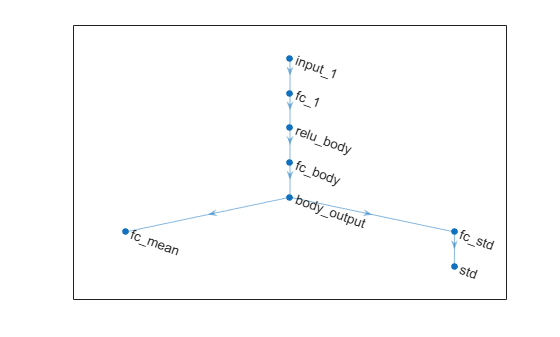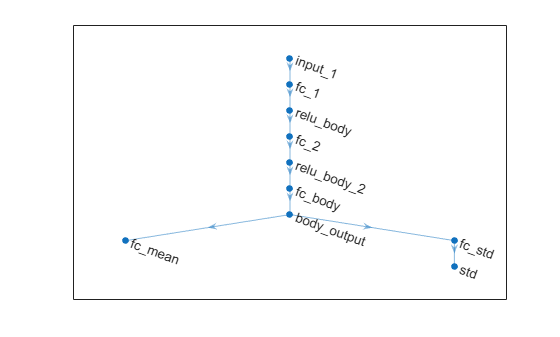Main Content

# getActor

Get actor representation from reinforcement learning agent

## Syntax

``actor = getActor(agent)``

## Description

example

````actor = getActor(agent)` returns the actor representation object for the specified reinforcement learning agent.```

## Examples

collapse all

Assume that you have an existing trained reinforcement learning agent. For this example, load the trained agent from Train DDPG Agent to Control Double Integrator System.

`load('DoubleIntegDDPG.mat','agent') `

Obtain the actor representation from the agent.

`actor = getActor(agent);`

Obtain the learnable parameters from the actor.

`params = getLearnableParameters(actor);`

Modify the parameter values. For this example, simply multiply all of the parameters by `2`.

`modifiedParams = cellfun(@(x) x*2,params,'UniformOutput',false);`

Set the parameter values of the actor to the new modified values.

`actor = setLearnableParameters(actor,modifiedParams);`

Set the actor in the agent to the new modified actor.

`agent = setActor(agent,actor);`

Create an environment with a continuous action space and obtain its observation and action specifications. For this example, load the environment used in the example Train DDPG Agent to Control Double Integrator System.

Load the predefined environment.

`env = rlPredefinedEnv("DoubleIntegrator-Continuous")`
```env = DoubleIntegratorContinuousAction with properties: Gain: 1 Ts: 0.1000 MaxDistance: 5 GoalThreshold: 0.0100 Q: [2x2 double] R: 0.0100 MaxForce: Inf State: [2x1 double] ```

Obtain observation and action specifications.

```obsInfo = getObservationInfo(env); actInfo = getActionInfo(env);```

Create a PPO agent from the environment observation and action specifications.

`agent = rlPPOAgent(obsInfo,actInfo);`

To modify the deep neural networks within a reinforcement learning agent, you must first extract the actor and critic representations.

```actor = getActor(agent); critic = getCritic(agent);```

Extract the deep neural networks from both the actor and critic representations.

```actorNet = getModel(actor); criticNet = getModel(critic);```

To view a network, use the `plot` function. For example, view the actor network.

`plot(actorNet)`You can modify the actor and critic networks and save them back to the agent. To modify the networks, you can use the Deep Network Designer app. To open the app for each network, use the following commands.

```deepNetworkDesigner(criticNet) deepNetworkDesigner(actorNet) ```

In Deep Network Designer, modify the networks. For example, you can add additional layers to your network. When you modify the networks, do not change the input and output layers of the networks returned by `getModel`. For more information on building networks, see Build Networks with Deep Network Designer.

To export the modified network structures to the MATLAB® workspace, generate code for creating the new networks and run this code from the command line. Do not use the exporting option in Deep Network Designer. For an example that shows how to generate and run code, see Create Agent Using Deep Network Designer and Train Using Image Observations.

For this example, the code for creating the modified actor and critic networks is in `createModifiedNetworks.m`.

`createModifiedNetworks`

Each of the modified networks includes an additional `fullyConnectedLayer` and `reluLayer` in their output path. View the modified actor network.

`plot(modifiedActorNet)`After exporting the networks, insert the networks into the actor and critic representations.

```actor = setModel(actor,modifiedActorNet); critic = setModel(critic,modifiedCriticNet);```

Finally, insert the modified actor and critic representations in the actor and critic objects.

```agent = setActor(agent,actor); agent = setCritic(agent,critic);```

## Input Arguments

collapse all

Reinforcement learning agent that contains an actor representation, specified as one of the following:

## Output Arguments

collapse all

Actor representation object, specified as one of the following:

## See Also

### Topics

Introduced in R2019a

Download ebook